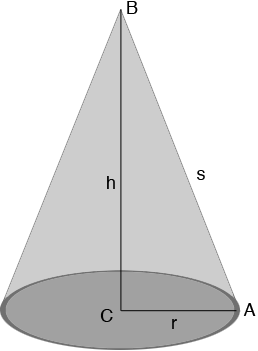SEARCH HOMEMath Central Quandaries & QueriesQuestion from Levi: While the mathematics at the welding shop page was very helpful there is one vital part missing. If I have never built a cone how do I figure out how much bigger the diameter of my circle has to be when laying flat on the floor verses the diameter when it has been pulled into a cone.Hi Levi,

Thanks for your kind words about our page about Mathematics at the Welding Shop. This was written by a grad 9 student in 1998. He is from Star City in Saskatchewan and went on a class visit to a welding shop. His task was to identify some of the mathematics he saw in the process of constructing cone shaped hopper bottoms for grain bins.The radius of the base is $r$ units, the height is $h$ units and the slant height is $s$ units. If you slice along the line $AB$ and roll it out flat then you have a sector of a circle of radius $s$ units. In your situation you know $r$ and $h$ and you want to find $s.$ The key is that triangle $ABC$ in the diagram above is a right triangle and hence you can use Pythagoras Theorem which says that

$|AB|^2 = |BC|^2 + |CA|^2$

or

$s^2 = h^2 + r^2$

Hence

$s = \sqrt{h^2 + r^2} \mbox{ units.}$

I hope this helps,
HarleyMath Central is supported by the University of Regina and the Imperial Oil Foundation.